# The Royal Consortium for DSP

## Noise Cancellation Project## Results and Discussion

WARNING - Due to the number of graphs and sound files, this page takes substantial time to load.

12/24/96

This section of our project report details the simulations we ran and the results we obtained from each simulation. We began with the simplest of experiments, in order to verify that we had a basic understanding of adaptive filters.

### Add 60 Hz "Noise" to a Recorded Voice

Using the Goldwave sound recording software, we recorded one second of speech, mono, at an 8K sample rate, for use in Matlab (student edition, and limited to 8,192 samples). We then generated a 60 Hz sine wave using Matlab and added the two signals together. By playing the summed signal, we could detect the 60 Hz overlaid on the voice. Note: We used an amplitude of unity for both the voice and the noise.

We processed the signal, using an adaptive filter of length 10, and an identical 60 Hz sine wave as the reference signal. Within a few samples, the sine wave was completely removed.

This experiment generated considerable discussion within the group. The most obvious question was: Why bother to use an adaptive filter when a notch filter would have worked at least as well? Second, what should the optimal filter length be? Third, how do we decide on a value for mu, the "convergence" factor?

To answer these questions, we tried a more complicated, "simple" simulation as described below.

### Filtering the Noise From a Tailpipe

We moved to the production version of Matlab and recorded about 15 seconds worth of tailpipe noise from a Chevrolet pickup truck. While recording, the engine speed was varied from a low idle to about 1500 RPM. For the first 10 seconds, the change in engine speed was slow and continuous, and during the last 5 second period, abrupt changes were made.Hear a Genuine Chevy Truck Below

Now Hear the Same Truck Through an LMS FIlter

As in the previous experiment, we used a filter length of 10 and used the noise signal as the reference signal. And as before, within a few samples, the noise was completely cancelled. The exception was where the engine speed changed rapidly. At these points we heard "popping" sounds.

We also varied mu in this experiment and discovered that if mu was too large, we quickly exceeded the maximum size of a floating point variable. A graph showed us that rather than converge, we began to oscillate about the desired point, with the oscillations growing. We determined (without any rigorous mathematical proof) that the filter length and the value of mu are inversely related when the filter weights are updated each sample. For a long filter, mu must be smaller.

This experiment provided several insights. First, the change in the noise must be relatively smooth, that is, no sharp changes. Upon review of the weighting algorithm, this makes sense, since it uses the gradient of the error function to compute new weights. Quick changes make for large derivative values.

More importantly, this experiment made us realize that our noise samples were too simplistic. In a real world muffler situation, the noise issued by the engine via the muffler and the noise as picked up by a microphone would differ in phase and frequency. This led us to try more the more complicated simulations described below.

### Recording the Electrical Responses of a Moving Muscle

In this simulation, we used sensors attached to the skin to record the electrical activity associated with muscle movement. The recorded signals however, were contaminated by a 60 Hz "hum" picked up by the sensors. An examination of the signal revealed that the noise was shifted in phase and varied in amplitude. We verified this fact by attempting to subtract a 60 Hz sine wave from the signal. It did not work.

INSERT GRAPH OF ELECTRICAL ACTIVITY AND ACTIVITY WITH SINE WAVE SUBTRACTED.

At last it appeared we had a real test of an adaptive filter which could not simply be mimiced by a notch filter, or by simply subtracting the noise from the signal. We ran our Matlab model again, using a filter length of 100. We used a 60 Hz sine wave with zero phase and a constant amplitude of one as the reference signal. We used a mu value of 0.02. Within a few samples the 60 Hz noise, even though it was shifted in phase and varied in amplitude, was almost completely removed.

INSERT GRAPH OF FILTERED ELECTRICAL ACTIVITY HERE

This struck us a quite an astounding result. The filter had managed to alter the fixed reference signal in such a way that it almost perfectly fit the noise in the signal. After much discussion, we began to gain insight into how the filter was actually operating. There are two ways of looking at the filter operation. Note that the discussion below applies to the removal of a single frequency.

First, each sample in a filter, excluding the first can be considered as a delay. Then, if one takes the simplest case of no phase change in the noise in the signal, the filter needs only adjust weights to create a notch filter for the frequency in question. Now, assume that there is a phase change in the noise in the input signal. The adaptive filter must have enough samples in it such that it can create a phase delay equal to the delay in the input signal. If the filter length is too short, or alternatively, if the phase change in the noise in the input signal is too large, the filter will not be able to remove the noise.

Another way of viewing this phenomenon is to consider that each sample in the frequency domain, when viewed in time, will be a sinc function, and the output signal will be the sum of all the sinc functions. There must be enough sinc functions present to sum and therefore fully recreate the signal.

Armed with this new insight into the operation of the adaptive filter, we decided to create simulations of the filter systems described earlier. We began with the Type I - System Identification filter scheme.

### Identifying the Impulse Response of an Unknown Plant

Actually, our plant impulse response was not unknown but instead was created with Matlab's ODE23 function which enabled us to specify a set of differential equations (as shown below) to create an analog system output.

GRAPH OF THE MATLAB ODE23 EQUATIONS HERE

Next, we fed the input signal (as shown below) into both the ODE23 "unknown plant" and the adaptive filter model.

GRAPH OF THE INPUT SIGNAL GOES HERE

We save the output respopnses of the ODE23 plant and the adaptive filter and then subtracted the two in order to determine the error. It can be seen from the graphs below that adaptive filter did a very good job of recreating the response of the unknown plant.

GRAPH OF IMPULSE RESPONSE HERE

### Type IV Systems - Noise Cancellation

Flushed with this kind of success, we decided to try a Type IV - Noise Cancellation System. We recorded a voice in mono at a 16K Hz sample rate for a period of 10 seconds. This signal was normalized to a maximum value of one. The voice graph and its wave file are shown below.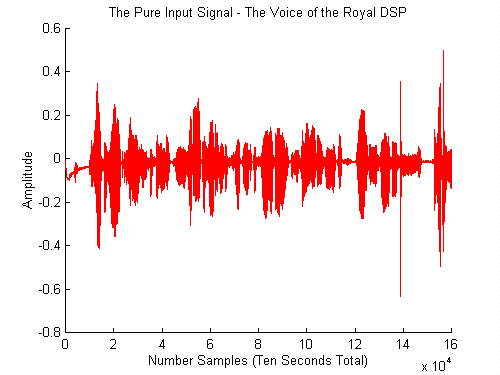Press the "Play" Button to Hear the Voice of the Royal DSP

After considerable discussion, we determined that "2 Live Crew", 'Bad As I Wanna Be' was indeed "noise" and therefore qualified to be used in this simulation. We extracted 16 seconds of the title track which became our official noise.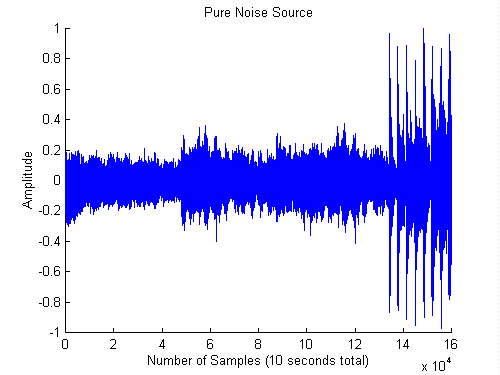Press "Play" and Hear the "Noise" for Yourself

From our previous simulations, we knew that a real test of our adaptive filter would require that our noise reference signal vary in phase and amplitude from the noise in the input signal. Thus, we passed the noise signal through a filter which had non linear phase and frequency response.

GRAPH OF FILTER FREQ AND PHASE

This produced a reference noise as shown below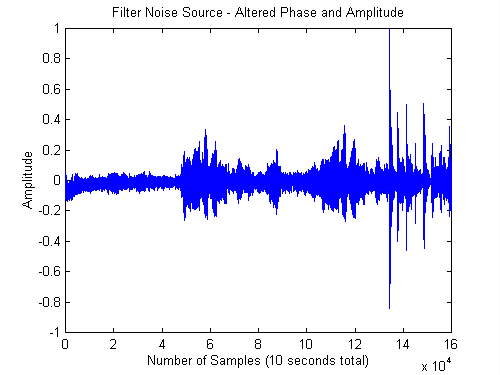See if 2 Live Crew Sounds Any Better Through a Filter

We normalized this noise to one, then doubled it so it had twice the maximum amplitude of the voice signal. Then we added the two together to create the input signal which can be seen and heard below. This became our input signal as shown and played below.The Voice of the Royal DSP Can't Be Heard for All the Racket!!

The obvious question was: Can we simply subtract the original noise from the input signal to retrieve the original voice. If so, then clearly, our reference signal did not differ enough from the input source to warrant the use of an adaptive filter. Our fears turned out to be unfounded: Simply subtracting the noise did not work, as demonstrated by the graphs and wave files below.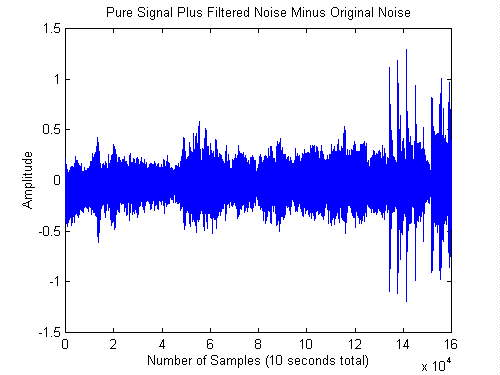Nope, 2 Live Crew Just Won't Go Away!

Finally, we ran our mixed voice signal through our adaptive filter. We used a filter length of 200 and our input and reference signals were each 160,000 samples long.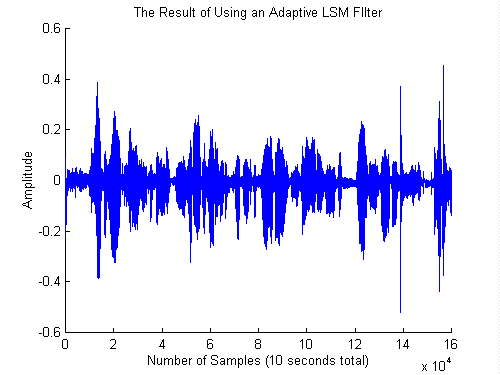Ahh, the Voice of the Royal DSP Has Been Restored

If you listen carefully, it is possible to hear the noise at the very beginning of the playback... then it quickly dies out as the filter converges to minimum power. Also, if one closely examines the graph and the recording, it can be noted that anywhere there is a very gross change in the noise (such as a symbol crash or strong bass drum), the noise is not completely removed. Nevertheless, the degree to which the "noise" has been removed from the input signal is substantial.

This computation turns out to be quite a challenge on a PC, even a Pentium 166. The fact that Matlab is an interpretive language did not help. In any event, after about 5 1/2 hours of processing, we had a new output signal. We were amazed! 2 Live Crew was almost totally absent. This can be verified from the graphs and wave files below.

### Block Weighted Adaptive Filters

The adaptive filter routine we used for all the simulations above updated the filter weights after each sample was processed. An adavantage of this method is that the filter converges rapidly inasmuch as weights are continuously adjusted. Caution must be exercised in the selection of mu, however. As the length of the filter increases, mu must be decreased or the possibility exists that the filter will "ring" or oscillate, rather than converge to minimum power. A disadvantage of this method is the computational resources required to update the weights after each sample.

Another alternative is the block adaptive method. The schematic for this type of weight updating is shown below.

SCHEMATIC OF BLOCK WEIGHT FILTER

When this method is used, the filter weights are updated every N samples where N is the length of the filter. The primary advantage of this method is increased computational efficiency. The disadvantage is slower convergence. Examples of a block weight filter as implemented in Matlab and Simulink are shown below.

CODE FOR BLOCK MATLAB FILTER HERE

CODE FOR SIMULINK STUFF HERE

10/31/96

Gee, Wally, I didn't know 120 dB would be hard on my hearing, even if it was phase shifted.

